## How to use the Cauchy-Schwarz inequality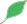This article is a stub.This means that it cannot be considered to contain or lead to any mathematically interesting information.

### Quick description

The Cauchy-Schwarz inequality asserts that on any measure space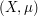, and any measurable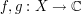, one has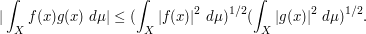Thus for instance one has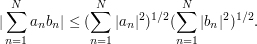This inequality is useful for decoupling an expression involving two functions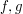, replacing that expression with one involving just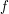, and one involving just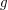. Only one of these latter expressions needs to be small (and the other one bounded) in order for the original expression to be small. Thus, one focus on estimating expressions involving just(say), effectively eliminatingfrom view.

### Example 1

Consider the following two ways of measuring the "size" of vectors in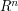. The-norm of a vector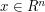is defined as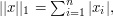and the-norm is defined as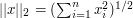.

What is the relationship between these two norms? It follows from the triangle inequality that the-norm is always bigger than the-norm. How much bigger?

The answer can be found through an application of the Cauchy-Schwarz inequality to the sequences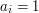and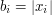: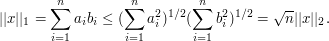Moreover, the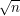factor in the above bound is the best possible: to see this plug in the vector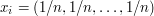.

### Example 2

(Counting 4-cycles in graphs)

### Example 3

(Some instance of the large sieve inequality)

### Example 4

(Suggestions welcome!)

### General discussion

The Cauchy-Schwarz inequality is efficient as long as you do expectandto behave in a roughly "parallel" manner. If instead they are behaving in an "orthogonal" manner then the inequality is quite lossy.

Another useful tool for decoupling is the Arithmetic-geometric mean inequality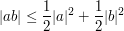or (slightly more generally)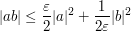for any complex numbers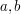, where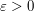is a parameter one can optimize in later.

(Discussion of converse Cauchy-Schwarz).

### Decoupling

In this article, "decoupling" sounds as an interesting general strategy. We could add a parent article about this more general metatechnique (I don't have the knowledge to elaborate it). Why is it important? In which cases ought we try to get a decoupling? Etc

Another "decoupling" technique that comes to mind now is that of "separating variables" in ODEs and PDEs.

### I agree, a lot more work is needed here

(Actually I am only now just beginning to realise the sheer scope of this project - it may end up making the Princeton Companion to Mathematics seem like a short story!) My strategy at this point is to lay down a large number of stubs and let them grow, develop, merge, etc. in unexpected ways, on the theory that the more articles already exist, the more likely it is that each potential contributor can find a niche.

I also want to write down a number of proofs of the Cauchy-Schwarz inequality here, as some of them emphasise some cute tricks (e.g. optimising in a parameter to be chosen later). I suppose initially we can put "How to use X" and "Proof of X" on the same page, though as discussed in the "different kind of article" thread we may eventually want to split them into two (interlinked) pages.

## Post new comment

(Note: commenting is not possible on this snapshot.)

Before posting from this form, please consider whether it would be more appropriate to make an inline comment using the Turn commenting on link near the bottom of the window. (Simply click the link, move the cursor over the article, and click on the piece of text on which you want to comment.)